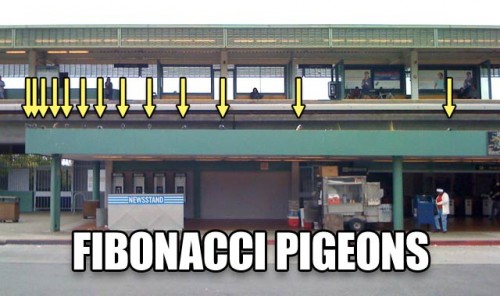# Brute forces perhapsI guess you all familiar with the Fibonacci sequence.

For those who haven't known this term yet, the Fibonacci sequence is a sequence of numbers which $F(0)=0$, $F(1)=1$ and for $n \ge 2$, $F(n)=F(n-1)+F(n-2)$.

So, here's my question:

If $F(x)$ is the largest prime Fibonacci number where $x \le 200$, find $\left\lfloor \log _{ x }{ F(x) } \right\rfloor$.

×